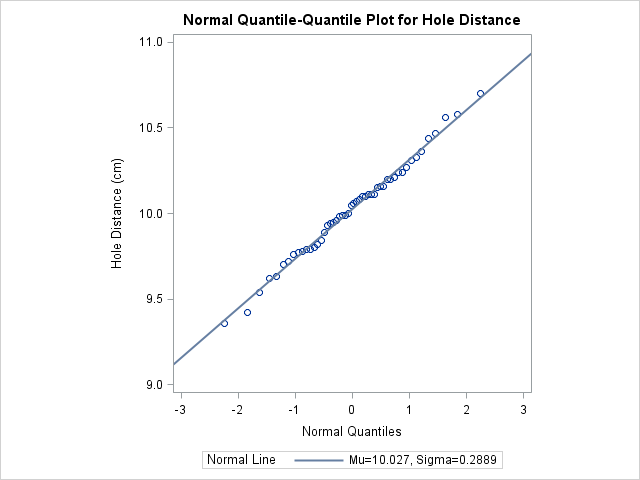# The UNIVARIATE Procedure

### Example 4.29 Adding a Distribution Reference Line

This example, which is a continuation of Example 4.28, illustrates how to add a reference line to a normal Q-Q plot, which represents the normal distribution with mean and standard deviation . The following statements reproduce the Q-Q plot in Output 4.28.1 and add the reference line:

```title 'Normal Quantile-Quantile Plot for Hole Distance';
proc univariate data=Sheets noprint;
qqplot Distance / normal(mu=est sigma=est)
odstitle = title
square;
run;
```

The plot is displayed in Output 4.29.1.

Specifying MU=EST and SIGMA=EST with the NORMAL primary option requests the reference line for which and are estimated by the sample mean and standard deviation. Alternatively, you can specify numeric values for and with the MU= and SIGMA= secondary options. The COLOR= and L= options specify the color and type of the line, and the SQUARE option displays the plot in a square format. The NOPRINT options in the PROC UNIVARIATE statement and after the NORMAL option suppress all the tables of statistical output produced by default.

Output 4.29.1: Adding a Distribution Reference Line to a Q-Q PlotThe data clearly follow the line, which indicates that the distribution of the distances is normal.

A sample program for this example, uniex17.sas, is available in the SAS Sample Library for Base SAS software.